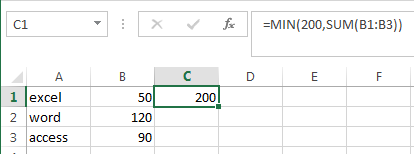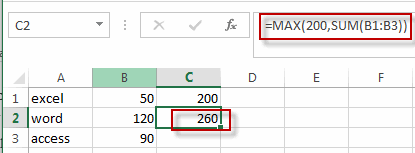# Limit Formula Result to Maximum or Minimum Value

This post will guide you how to limit formula result to a maximum or minimum value in excel. For example, if you want to limit the results of a formula to a maximum value of 200, how to this this in excel. How do I return a formula result only within a specified minimum or maximum values in excel. Do we have any ways to limit the results of a calculation to a maximum value.

You can use the MIN function or Max function to create a formula to limit a number within a minimum or maximum value. Just do the following steps:

## Limit Formula Result to Maximum Value

If you want to limit a formula result to a maximum value 200, you can use the MIN function to create a formula, just like this:

```=MIN(200,SUM(B1:B3))```

If the result of SUM function is greater than 200, then the MIN function will return value 200. Otherwise, return the result of SUM function.

## Limit Formula Result to Minimum value

If you want to limit a formula result (for example, the sum function) to a minimum value, then you can use the MAX function in combination with the SUM function or other function to create a new formula. Just like this:

`=MAX(200,SUM(B1:B3))`
``

If the result returned by the SUM function is smaller than 200, then returns value 200, otherwise, it will return the result returned by the SUM function.

### Related Functions

• Excel SUM function
The Excel SUM function will adds all numbers in a range of cells and returns the sum of these values. You can add individual values, cell references or ranges in excel.The syntax of the SUM function is as below:= SUM(number1,[number2],…)…
• Excel MIN function
The Excel MIN function returns the smallest numeric value from the numbers that you provided. Or returns the smallest value in the array.The MIN function is a build-in function in Microsoft Excel and it is categorized as a Statistical Function.The syntax of the MIN function is as below:= MIN(num1,[num2,…numn])….
• Excel MAX function
The Excel MAX function returns the largest numeric value from the numbers that you provided. Or returns the largest value in the array.= MAX(num1,[num2,…numn])…

Related Posts

6 Best Free Wedding Budget Templates

This post will show you some free wedding budget spreadsheet templates for both google sheets and Microsoft Excel Spreadsheet, as well as some explanations of the features or important function points of these templates. You can then easily make modifications ...

Calculate Cumulative Totals with Excel SUM Function

Today, through a simple example, we will show you how to use one of the most common-used Mathematical functions in excel, the SUM function, to add up the sum. In our daily life, we keep an account of what we ...

Check Dates in chronological order

Assume you have a date list that has different date formats, as seen in the accompanying picture. In this instance, Excel's Sort function will fail to sort them appropriately. However, you may convert all various date formats to a particular ...

How to Use 3D SUM Multiple Worksheets

To sum a range of numbers is straightforward for most Excel users, but do you know how to establish a 3D reference to total the same range of numerous sheets. In this post, I will present the steps for this ...

If Cell Begins with One of Three Supplied Characters

If you want to test values to see if they begin with some given specific characters like “x”, ”y”, or “z”, you can create a formula with COUNTIF and SUM functions to return results. EXAMPLE You can see “TRUE” or ...

Average per Week by Formula in Excel

We usually apply AVERAGE function or relevant functions to return average directly in Excel worksheet. But in some situations, only applying average relevant functions cannot figure out our problem. Sometimes we can create a formula with functions and mathematical operation ...

How to Sum if Contains an Asterisk

To add numbers together we need to apply SUM function. And if we want to add numbers based on some conditions, we can add criteria with the help of SUMIFS function, SUMIFS can filter data with multiple criteria effectively. If ...

How to Sum in Vertical Range

If we want to add numbers based on some conditions in Excel worksheet, we can add criteria with the help of SUMIFS function, SUMIFS can filter data with multiple criteria effectively. In this article, we will introduce you the method ...

How to Sum in Horizontal Range

To add numbers together we need to apply SUM function. But if we want to add numbers based on some conditions, we can add criteria with the help of SUMIFS function, SUMIFS can filter data with multiple criteria effectively. In ...

How to Sum with Criteria and Or Logic in Excel

To add numbers together we need to apply SUM function. And if we want to add numbers based on some conditions, we can add criteria with the help of SUMIFS function, SUMIFS can filter data with multiple criteria effectively. If ...

Sidebar